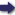(London :  Kegan Paul, Trench, Trübner & Co.,  1910.)

 Tools

## Search this bookPrev Page 36 Next``` 2,6 ALBERUNTS INDIA. [Lacuna.) and the number they get is subtracted from the num¬ ber written down in the middle place. The double of the remainder they divide by 65. Then the quotient represents the partial adhimdsa months. This number they add to that one which is written down in the uppermost place. They multiply the sum by 30, and add to the product the days which have elapsed of the current month. The sum represents the partial solar days. This number is written down in two different places, one under the other. They multiply the lower number by ii, and write the product under it. Then they divide it by 403,963, and add the quotient to the middle number. They divide the sum by 703, and the quotient represents the partial dnccrdtrct days. This number they subtract from the number written in the uppermost place, and the remainder is the number of civil days which we want to find." Explication The rationale of this computation is the following:— method. If WO divide the universal solar months by the uni¬ versal adhimdsa months, we get as the measure of one adhimdsa month Z'^^i'^'^ii solar months. The double of this is 65Yy-9%% solar months. If we divide by this number the double of the months of the given years, the quotient is the.number of the partial ctdhimdsas. How¬ ever, if we divide by wholes plus a fraction, and want to subtract from the number which is divided a certain portion, the remainder being divided by the wholes only, and the two subtracted portions being equal por¬ tions of the wholes to which they belong, the whole divisor stands in the same relation to its fraction as the divided number to the subtracted portion. The latter If wc make this computation for our gauge-year, we ^piiedto get the fraction of T,irVV/tfo^"o> ^.nd dividing both num- the gauge- -, -, _ i. n i year. bcTS by 15, wc get WtW- It would also be possible here to reckon by single adhimdsas instead of double ones, and in that case it ```Prev Page 36 Next